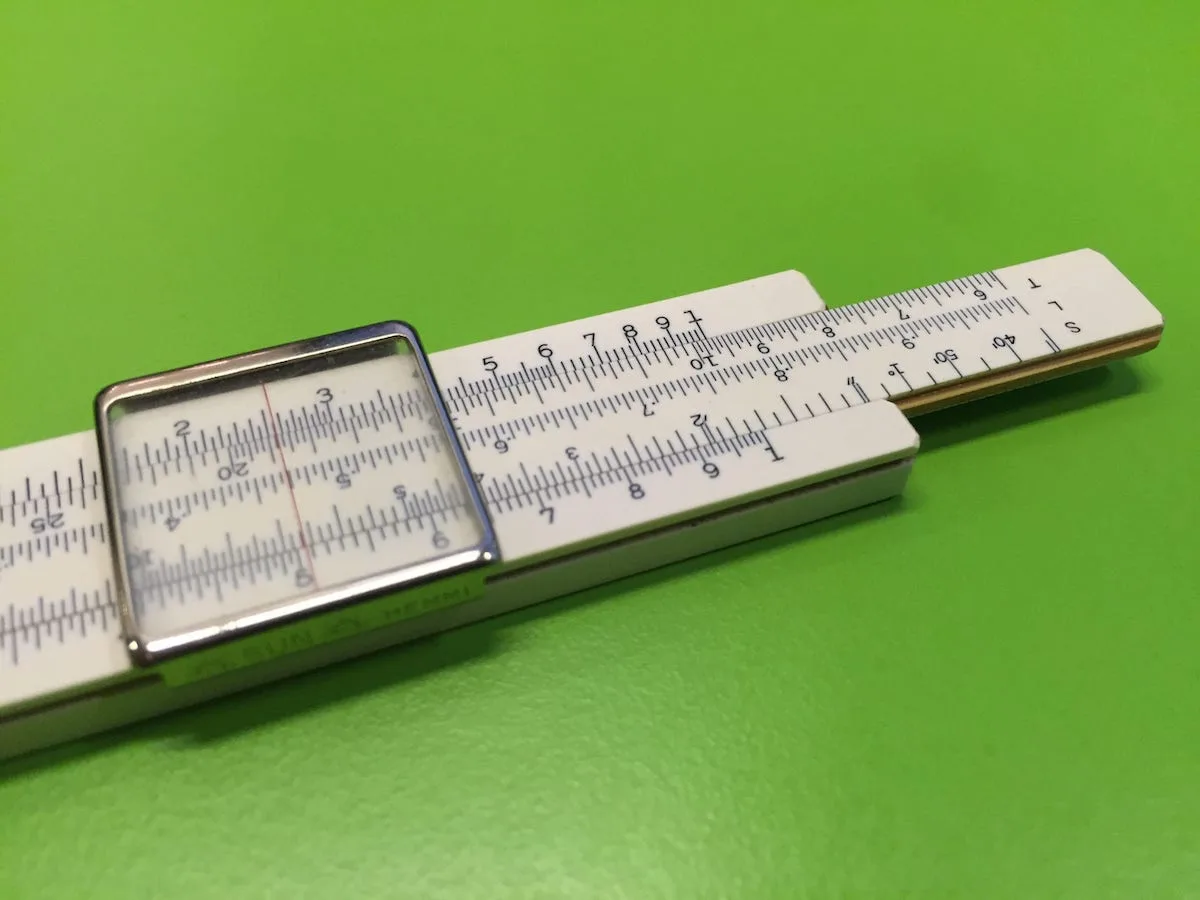# Automotive Conversions You Need to KnowNeed to figure out how much a gallon of gas weighs? How about metric to SAE, or what displacement that junk yard engine you found is? Check out our handy conversions, 38 in total, to make it easy to figure all this out.

### Quick Conversions

Ounces vs. Grams: 1 ounce = 28.35 grams
Pounds vs. Grams: 1 pound = 453.6 grams
Pounds vs. Ounces: 1 pound = 16 ounces
Inch vs. Centimeter: 1 inch = 2.540 cm
Inch vs. Millimeter: 1 inch = 25.40 mm
Gallons of Nitro to Pounds: 1 gallon of nitro = 9.5 pounds/1 quart = 2.37 pounds
Gallons of Alcohol to Pounds: 1 gallon of alcohol = 6.6 pounds/1 quart = 1.65 pounds
Gallons of Gasoline to Pounds: 1 gallon of gasoline = 6.2 pounds/1 quart = 1.55 pounds
Gallons of Water to Pounds: 1 gallon of water = 8.3 pounds/1 quart = 2.08 pounds
Gallons of Oil to Pounds: 1 gallon of oil = 7 pounds/1 quart = 1.75 pounds

### Metric and Standard Conversions

Kilometers to Feet: 1 kilometer = 1,000 meters = 3,280.83 feet
Kilometers to Miles: 1 kilometer = 1,000 meters = .6215 mile
Meters to Inches: 1 meter = 100 centimeters = 39.37 inches
Meters to Yards: 1 meter = 100 centimeters = 1.09 yards
Decimeter to Inches: 1 decimeter = 3.937 inches
Centimeter to Inches: 1 centimeter = .3937 inches
Kilograms to Pounds: kilograms/2.20 = pounds
Pounds vs. Grams: 1 pound = 453.6 grams
Ounces vs. Grams: 1 ounce = 28.35 grams
Grams vs. Millgrams: 1 gram = 1,000 milligrams

### Engine and Performance

Cubic Inches (CID) = CC ÷ 16.39
Cubic Centimeters (CC) = CID x 16.39
Lbs/hr. (LPH) = divide cc/min by 10.5
Gallons/hr. (GPH) = divide lbs/hr. by 6.0

### Automotive Plumbing

-AN Size to Fractional Equivalent

-3 AN = 3/16″
-4 AN = 1/4″
-6 AN = 3/8″
-8 AN = 1/2″
-10 AN = 5/8″
-12 AN = 3/4″
-16 AN = 1″
-20 AN = 1-1/4″
-24 AN = 1-1/2″

Cheat sheets have a bad connotation attached to them. They were definitely frowned upon in school and not always accepted in the workplace. With so many equations and formulas to remember in the auto shop having thes eon hand can be a bi time saver. Copy it to your phone, print it if you feel old school or just bookmark it on that CFT screen shop computer that runs AOL dial up.

### Racing Carburetor CFM

Racing Carburetor CFM = RPM x Displacement ÷ 3456 x 1.1

### Displacement

Displacement = .7854 x Bore2 x Stroke x Number of Cylinders

### Correct Compression Ratio (CCR)

CCR = FCR (Altitude/1,000) x .2

### Tire Diameter

Tire Diameter = (MPH x Gear Ratio x 336) ÷ RPM

### Rocker Arm Ratio and Valve Lift

Gross Valve Lift = Camshaft Lobe Lift x Rocker Arm Ratio

### Horsepower

Horsepower = (RPM x Torque) ÷ 5,252

### Torque

Torque = (5,252 x HP) ÷ RPM

### Rod Ratio

Rod Ratio = Rod Length ÷ Crank Stroke Length

### Average Piston Speed

Average Piston Speed = Crank Stroke x RPM ÷ 6

### Rear Gear Ratio

Rear Gear Ratio = (RPM at Finish Line x Tire Diameter) ÷ (MPH x 336)
Note: You can also save this link to a handy Gear Ratio calculator.

### Volume (CCs) of Deck Clearance

CCs of Deck Clearance = Bore x Bore x 12.87 x Depth of Deck Clearance